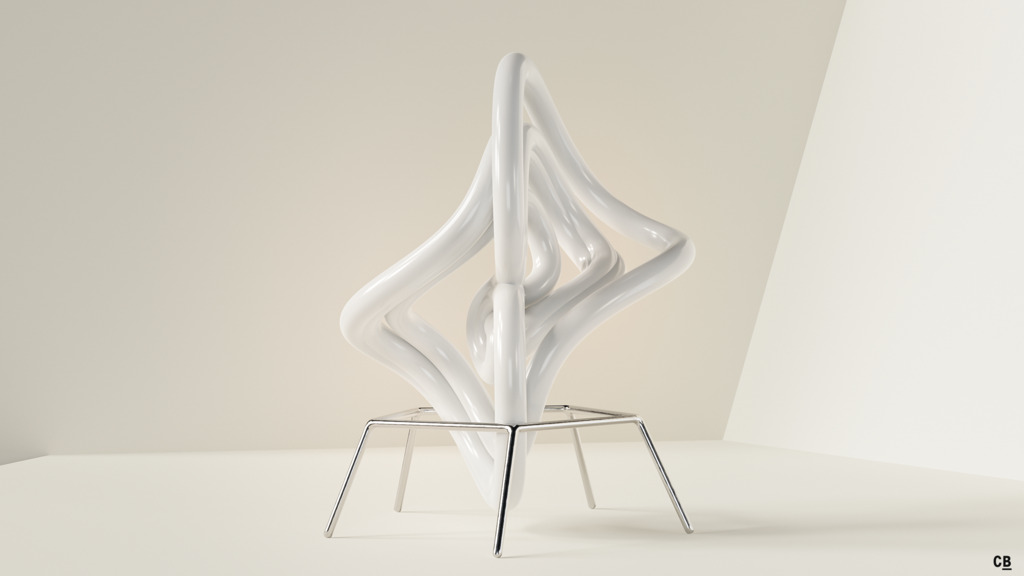# Qi-Chen Attractor

As several other systems like Rössler, Chen or Lü, this attractor found by Guoyuan Qi and his colleagues1 in 2005 is derived from the famous Lorenz equations.

The original set is:

$\dot{x} = a\,(y - x) + y\,z$ $\dot{y} = c\,x - y - x\,z$ $\dot{z} = b\,z + x\,y$

On the internet one can find a version with slightly modified constants. It seems to be referred to as the “Sakarya attractor”, but should be named after Qi et al. who found the system. Please contact me, if you happen to know more about the naming confusion.

## Renders

Differential system:

$\dot{x} = y - x + y\,z$ $\dot{y} = -x - y - a\,x\,z$ $\dot{z} = z - b\,x\,y$

Constants:

$a = 0.82$ $b = 0.78$1. G. Qi, G. Chen, S. Du, Z. Chen and Z.Yuan, 2005. "Analysis of a new chaotic system". Phys. A. 352(295–308). doi:10.1016/j.physa.2004.12.040

🕸 💍